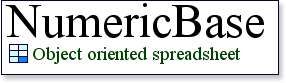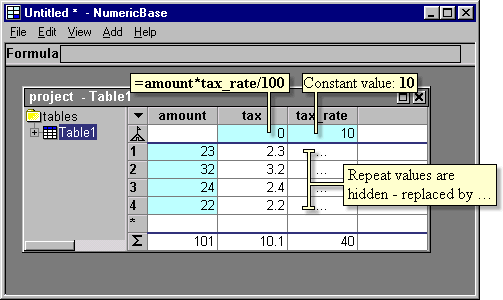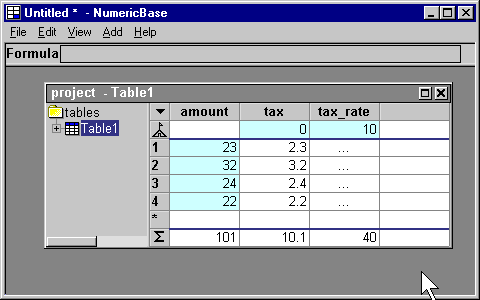# Hide Repeating values

hide repeating values.
If the formula at the base row is a constant value that does not change across the rows, then NumericBase only shows the value at the base row. All the values at the rows are replaced by "..." string. This is useful to give solutions a less cluttered appearance.

In the following screenshot, we have a column called tax_rate. This column has a formula at the base row that is a constant value: 10. because the formula at the base row is just a constant, the values at the data rows are hidden and replaced by three dots (...).screenshot: hide repeating values.

Overriding a constant value
If you override the constant value, then NumericBase no longer hides the values - see example in the following animated screenshot.Animated screenshot: Overriding a constant value.